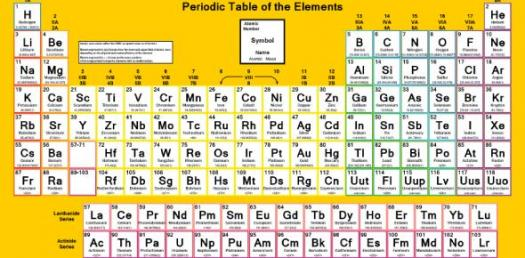# Periodic Table And Basic Chemistry

30 Questions | Total Attempts: 543SettingsElements are different and what bring about the difference are their atomic numbers and sizes. Solids, liquids and gases are all elements but have been grouped differently in the periodic table based on their atomic sizes. Their atomic numbers and sizes are what give these elements their properties. Test your basic knowledge of chemistry by taking the quiz.

• 1.
A vertical column on the periodic table is called a
• A.

Period

• B.

Group or family

• C.

Transition

• D.

Metal

• 2.
A horizontal row on the periodic table is called a
• A.

Period

• B.

Group or family

• C.

Metal

• D.

Metalloid

• 3.
Most of the elements oin the periodic table are classified as...
• A.

Nonmetals

• B.

Gases

• C.

Metals

• D.

Metalloids

• 4.
The elements that touch the zigzag line are classified as...
• A.

Metals

• B.

Nonmetals

• C.

Metalloids

• D.

Liquids

• 5.
Elements in the first group are extremely reactive and are referred to as...
• A.

Alkali metals

• B.

Alkaline Earth metals

• C.

Halogens

• D.

Noble Gases

• 6.
Elements in groups 3 through 12 are not as predictable as the other metals but are some of the best known metals.  They are called the ....
• A.

Alkaline Earth metals

• B.

Lanthanide series

• C.

Transition metals

• D.

Halogens

• 7.
This family of elements are unreactive because they have 8 valence electrons already. They are the ...
• A.

Halogens

• B.

Noble Gases

• C.

Transition metals

• D.

Boron family

• 8.
The modern Periodic Table is organized by ...
• A.

Increasing atomic number

• B.

Increasing atomic mass

• C.

Increasing number of electron shells

• D.

Alphabetical order

• 9.
The first Periodic Table was organized by increasing atomic mass by this man.
• A.

Newton

• B.

Einstein

• C.

Mendeleev

• D.

Hawking

• 10.
By finding an element on the periodic table you can predict...
• A.

It's reactivity

• B.

It's basic properties

• C.

The number of valence electrons

• D.

• 11.
Isotopes of elements have the same number of protons but different numbers of...
• A.

Neutrons

• B.

Electrons

• C.

Valence electrons

• D.

Energy levels

• 12.
What does AMU stand for?
• A.

Atoms met up

• B.

Atomic mass unit

• C.

Number of protons

• D.

Number of electrons

• 13.
Which of the following would have 7 valence electrons?
• A.

Flourine

• B.

Lithium

• C.

Aluminum

• D.

Potassium

• 14.
Which of the following would be the most stable element?
• A.

Lithium

• B.

Barium

• C.

Chlorine

• D.

Argon

• 15.
Copper, zinc, and iron are all considered
• A.

Transition metals

• B.

Alkali metals

• C.

Halogens

• D.

Alkaline earth metals

• 16.
I have 35 protons in my nucleus...who am I?
• A.

Lithium

• B.

Sodium

• C.

Bromine

• D.

Krypton

• 17.
I have 6 protons, 6 electrons, and 6 neutrons...who am I?
• A.

Helium

• B.

Iodine

• C.

Carbon

• D.

Calcium

• 18.
This element doesn't really belong to any family.  It has one valence electron.  It is special and usually in a square on the left side of the table above the first family.
• A.

Helium

• B.

Hydrogen

• C.

• D.

Cobalt

• 19.
This element has 87 electrons in a neutral atom.
• A.

Lithium

• B.

Francium

• C.

Phosphorus

• D.

Carbon

• 20.
The number of protons plus the average number of neutrons in this element is 9.0.
• A.

Hydrogen

• B.

Lithium

• C.

Beryllium

• D.

Nitrogen

• 21.
This sub atomic particle is positive and found in the nucleus of an atom.
• A.

Proton

• B.

Neurtron

• C.

Electron

• D.

Valence electron

• 22.
This sub atomic particle is negative and found in the "cloud" around the nucleus of an atom.
• A.

Proton

• B.

Neutron

• C.

Electron

• D.

Valence electron

• 23.
These elements are used in computers and electronics because of their ability to be semi-conductors.
• A.

Transition metals

• B.

Metalloids

• C.

Nonmetals

• D.

Noble gases

• 24.
If I am an element that is ductile and malleable, I am probably...
• A.

A metal

• B.

A nonmetal

• C.

A metalloid

• D.

A gas

• 25.
If I am an element that is not conductive of heat or electricity, and I am brittle and dull, I am probably a...
• A.

Metal

• B.

Nonmetal

• C.

Gas

• D.

Liquid

Related TopicsBack to top